# AP Statistics : Defining Errors

## Example Questions

### Example Question #1 : Ap Statistics

If a test has a power of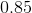, what is the probability of Type II error?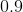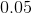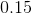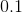Explanation:

From the statistical definition of power (of a test), the power is equal to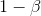where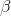represents the Type II error.

Therefore our equation to solve becomes: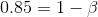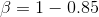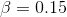### Example Question #1 : How To Define A Type Ii Error

You and a classmate wanted to test the effect of sugars and fats on levels of blood sugar.

Your classmate told you that they found the null hypothesis valid, which was what there is no difference between the effects of sugars and fats on blood sugar levels.

If the null hypothesis was actually false, what type of error was made?

Neither

Type I

Type I and II

Type II

Type II

Explanation:

A type I error occurs when the null hypothesis is valid but rejected.

A type II error occurs when the null hypothesis is false, but fails to be rejected.

Because the null hypothesis was false, but had failed to be rejected, they made a Type II error.

### Example Question #1 : How To Define A Type Ii Error

You and a friend wanted to test the effect of similar servings of juice and soda on blood sugar levels.

Your friend told you that they found the null hypothesis valid, which was what there is no difference between the effects of similar servings of juice and soda on blood sugar levels.

If the null hypothesis was actually false, what type of error was made?

Type I

Type II

Neither

Type I and II

Type II

Explanation:

A type I error occurs when the null hypothesis is valid but rejected.

A type II error occurs when the null hypothesis is false, but fails to be rejected.

Because the null hypothesis was false, but had failed to be rejected, they made a Type II error.

### Example Question #11 : Inference

A factory claims that only 1% of their widgets are defective but a large amount of their produced widgets have been breaking for customers.  A test is conducted to figure out if the factory claim of 1% defective is true or if the customers claim of graeater than 1% is true.  What would be an example of a Type II error?

The test shows that only 1% are defective when the truth is that more than 1% are defective.  The null is upheld when it should be rejected.

The test shows that there are more than 1% defective even though the null of just 1% is actually true.

The test shows that the percentage of defective widgets is 1% and the factory claim is upheld.

The test came up with no definitive answer.

More than 1% are shown to be defective and the reject the factory claim of only 1% defective.

The test shows that only 1% are defective when the truth is that more than 1% are defective.  The null is upheld when it should be rejected.

Explanation:

Type II Error is not rejecting a truly false null hypothesis.  This means that the test supports the factory claim of 1% even though the true amount is more than that.

### Example Question #1 : How To Define A Type I Error

A prominent football coach is being reviewed for his performance in the past season. To evaluate how well the coach has done, the team manager runs a statistical test comparing the coach to a sample of coaches in the league. If the test suggests that the coach outperformed other coaches when in fact he did not, and the manager then rejects the null hypothesis (that the coach did not outperform the other coaches), what kind of error is he committing?

Type M error

None of the above

Type II error

Type I error

Type I error

Explanation:

A type I error occurs when one rejects a null hypothesis that is in fact true. The null hypothesis is that the coach does not outperform other coaches, and the test reccomends that we reject it even though it is true. Thus, a type I error has been committed.

### Example Question #1 : How To Define A Type I Error

If a hypothesis test uses a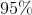confidence level, then what is its probability of Type I Error?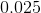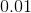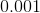Explanation:

By definition, the probability of Type I Error is,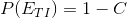where,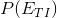represents Probability of Type I Error andrepresents the confidence level.

Thus resulting in: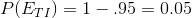### Example Question #1 : Defining Errors

For significance tests, which of the following is an incorrect way to increase power (the probability of correctly rejecting the null hypothesis)?

Increase sample size

Decrease the probability of making a Type I error

Increase the difference between the null hypothesis and the alternative hypothesis

Decrease variability of samples

Increase the probability of making a Type I error

Decrease the probability of making a Type I error

Explanation:

Recall that power is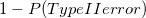. The probability of Type I and Type II errors will change inversely of each other as the probability of making a Type I error changes. If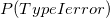increases, then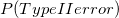decreases, and as a result power will increase. So ifdecreases,would increase, and power would decrease; therefore decreasingwill not increase power.

### Example Question #1 : How To Define A Type I Error

In a recent athletic study, your lab partner told you that they rejected the null hypothesis that the fabric of running shoes has no effect on the wearer's running times.

If the null hypothesis was actually valid, what type of error was made?

Type II

Type I

Neither

Type I and II

Type I

Explanation:

A type I error occurs when the null hypothesis is valid but rejected.

A type II error occurs when the null hypothesis is false, but fails to be rejected.

Because the null hypothesis was true, but rejected, they made a Type I error.

### Example Question #2 : How To Define A Type I Error

In a recent academic study, your lab partner told you that they rejected the null hypothesis that the ionization of water has no effect on the rate of grass growth.

If the null hypothesis was actually valid, what type of error was made?

Type II

Type I and II

Neither

Type I

Type I

Explanation:

A type I error occurs when the null hypothesis is valid but rejected.

A type II error occurs when the null hypothesis is false, but fails to be rejected.

Because the null hypothesis was true, but rejected, they made a Type I error.

### Example Question #1 : How To Define A Type I Error

A company claims that they have 12 ounces of potato chips in each of their bags of chips.  A customer complaint is filed that they do not truly contain 12 ounces but actually contain less.  A sampling test is conducted to see if the comapny measure is true or not. What would be an example of a Type I error?

There test does not come up with a defined answer.

The test finds that there are 12 ounces in each but the true amount is less than that.

There are 12 ounces in each bag and the test backs the company claim.

The test finds that there is less than 12 ounces and the customer claim was indeed true.

The test finds there are less than 12 ounces but there are actually 12 ounces.